# 二、使用步骤

## 1.数据预处理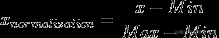对于指标来说，一般分有正向指标和负向指标之分：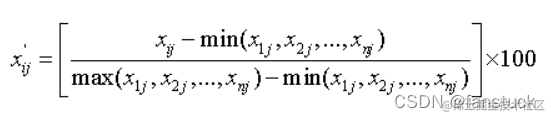负向指标：指标值越大评价越差的指标，如死亡率，失业率。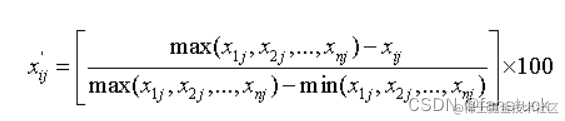## 2.计算指标信息熵

（1）计算第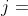项指标下第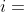个样本值占比重：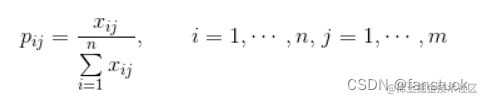(2) 计算各指标的信息熵：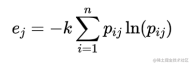其中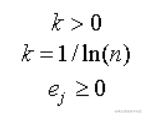项指标的数据差异越大，熵值越小；反之，熵值越大。

(3)计算信息效用值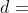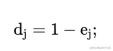(4)计算指标权重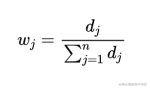## 3.相关代码

``` import numpy as np import pandas as pd data = pd.read_csv(r"C:\Users\指标.csv",sep=',') data.info() data

# 标准化数据

data_test1=data.iloc[:,1:5] data_test1 data_test2 = (data_test1 - data_test1.min())/(data_test1.max() -data_test1.min()) data_test2 m,n=data_test2.shape

# 将dataframe格式转化为matrix格式

data_test3=data_test2.values data_test3 k=1/np.log(m) yij=data.sum(axis=0) pij=data/yij

# 第二步，计算pij

test=pij*np.log(pij) test=np.nan_to_num(test)

# 计算每种指标的信息熵

ej=-k*(test.sum(axis=0))

# 计算每种指标的权重

wi=(1-ej)/np.sum(1-ej)

```

# 三、实例运用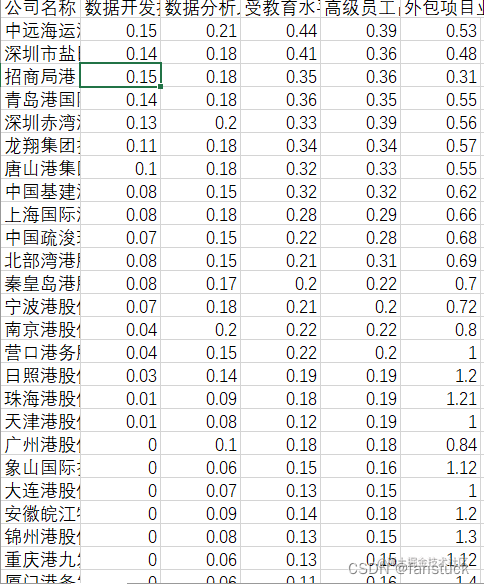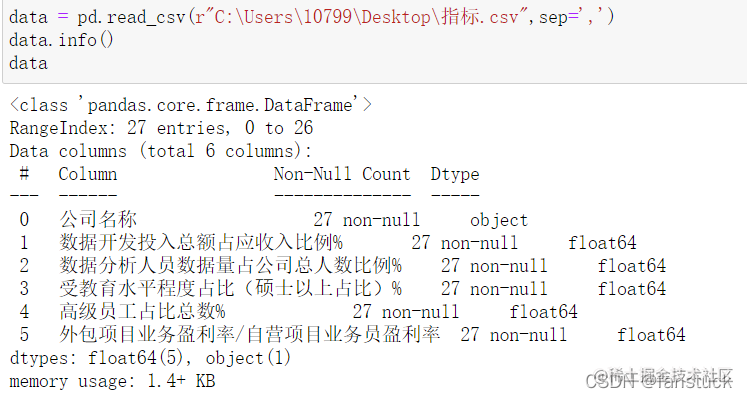# 四、总结

## 优点

• 能深刻反映出指标的区分能力，进而确定权重
• 是一种客观赋权法，相对主管赋权具有较高的可信度和精确度
• 算法简单

## 缺点

• 不够智能，没有考虑指标与指标之间的影响，如：相关性、层级关系等
• 若无业务经验指导，权重可能失真
• 对样本的依赖性较大，随着建模样本不断变化，权重会发生一定波动